# sklearn.linear_model.Ridge¶

class sklearn.linear_model.Ridge(alpha=1.0, *, fit_intercept=True, copy_X=True, max_iter=None, tol=0.0001, solver='auto', positive=False, random_state=None)[source]

Linear least squares with l2 regularization.

Minimizes the objective function:

||y - Xw||^2_2 + alpha * ||w||^2_2


This model solves a regression model where the loss function is the linear least squares function and regularization is given by the l2-norm. Also known as Ridge Regression or Tikhonov regularization. This estimator has built-in support for multi-variate regression (i.e., when y is a 2d-array of shape (n_samples, n_targets)).

Read more in the User Guide.

Parameters:
alpha{float, ndarray of shape (n_targets,)}, default=1.0

Constant that multiplies the L2 term, controlling regularization strength. alpha must be a non-negative float i.e. in [0, inf).

When alpha = 0, the objective is equivalent to ordinary least squares, solved by the LinearRegression object. For numerical reasons, using alpha = 0 with the Ridge object is not advised. Instead, you should use the LinearRegression object.

If an array is passed, penalties are assumed to be specific to the targets. Hence they must correspond in number.

fit_interceptbool, default=True

Whether to fit the intercept for this model. If set to false, no intercept will be used in calculations (i.e. X and y are expected to be centered).

copy_Xbool, default=True

If True, X will be copied; else, it may be overwritten.

max_iterint, default=None

Maximum number of iterations for conjugate gradient solver. For ‘sparse_cg’ and ‘lsqr’ solvers, the default value is determined by scipy.sparse.linalg. For ‘sag’ solver, the default value is 1000. For ‘lbfgs’ solver, the default value is 15000.

tolfloat, default=1e-4

Precision of the solution. Note that tol has no effect for solvers ‘svd’ and ‘cholesky’.

Changed in version 1.2: Default value changed from 1e-3 to 1e-4 for consistency with other linear models.

solver{‘auto’, ‘svd’, ‘cholesky’, ‘lsqr’, ‘sparse_cg’, ‘sag’, ‘saga’, ‘lbfgs’}, default=’auto’

Solver to use in the computational routines:

• ‘auto’ chooses the solver automatically based on the type of data.

• ‘svd’ uses a Singular Value Decomposition of X to compute the Ridge coefficients. It is the most stable solver, in particular more stable for singular matrices than ‘cholesky’ at the cost of being slower.

• ‘cholesky’ uses the standard scipy.linalg.solve function to obtain a closed-form solution.

• ‘sparse_cg’ uses the conjugate gradient solver as found in scipy.sparse.linalg.cg. As an iterative algorithm, this solver is more appropriate than ‘cholesky’ for large-scale data (possibility to set tol and max_iter).

• ‘lsqr’ uses the dedicated regularized least-squares routine scipy.sparse.linalg.lsqr. It is the fastest and uses an iterative procedure.

• ‘sag’ uses a Stochastic Average Gradient descent, and ‘saga’ uses its improved, unbiased version named SAGA. Both methods also use an iterative procedure, and are often faster than other solvers when both n_samples and n_features are large. Note that ‘sag’ and ‘saga’ fast convergence is only guaranteed on features with approximately the same scale. You can preprocess the data with a scaler from sklearn.preprocessing.

• ‘lbfgs’ uses L-BFGS-B algorithm implemented in scipy.optimize.minimize. It can be used only when positive is True.

All solvers except ‘svd’ support both dense and sparse data. However, only ‘lsqr’, ‘sag’, ‘sparse_cg’, and ‘lbfgs’ support sparse input when fit_intercept is True.

New in version 0.17: Stochastic Average Gradient descent solver.

New in version 0.19: SAGA solver.

positivebool, default=False

When set to True, forces the coefficients to be positive. Only ‘lbfgs’ solver is supported in this case.

random_stateint, RandomState instance, default=None

Used when solver == ‘sag’ or ‘saga’ to shuffle the data. See Glossary for details.

New in version 0.17: random_state to support Stochastic Average Gradient.

Attributes:
coef_ndarray of shape (n_features,) or (n_targets, n_features)

Weight vector(s).

intercept_float or ndarray of shape (n_targets,)

Independent term in decision function. Set to 0.0 if fit_intercept = False.

n_iter_None or ndarray of shape (n_targets,)

Actual number of iterations for each target. Available only for sag and lsqr solvers. Other solvers will return None.

New in version 0.17.

n_features_in_int

Number of features seen during fit.

New in version 0.24.

feature_names_in_ndarray of shape (n_features_in_,)

Names of features seen during fit. Defined only when X has feature names that are all strings.

New in version 1.0.

RidgeClassifier

Ridge classifier.

RidgeCV

Ridge regression with built-in cross validation.

KernelRidge

Kernel ridge regression combines ridge regression with the kernel trick.

Notes

Regularization improves the conditioning of the problem and reduces the variance of the estimates. Larger values specify stronger regularization. Alpha corresponds to 1 / (2C) in other linear models such as LogisticRegression or LinearSVC.

Examples

>>> from sklearn.linear_model import Ridge
>>> import numpy as np
>>> n_samples, n_features = 10, 5
>>> rng = np.random.RandomState(0)
>>> y = rng.randn(n_samples)
>>> X = rng.randn(n_samples, n_features)
>>> clf = Ridge(alpha=1.0)
>>> clf.fit(X, y)
Ridge()


Methods

 fit(X, y[, sample_weight]) Fit Ridge regression model. get_params([deep]) Get parameters for this estimator. Predict using the linear model. score(X, y[, sample_weight]) Return the coefficient of determination of the prediction. set_params(**params) Set the parameters of this estimator.
fit(X, y, sample_weight=None)[source]

Fit Ridge regression model.

Parameters:
X{ndarray, sparse matrix} of shape (n_samples, n_features)

Training data.

yndarray of shape (n_samples,) or (n_samples, n_targets)

Target values.

sample_weightfloat or ndarray of shape (n_samples,), default=None

Individual weights for each sample. If given a float, every sample will have the same weight.

Returns:
selfobject

Fitted estimator.

get_params(deep=True)[source]

Get parameters for this estimator.

Parameters:
deepbool, default=True

If True, will return the parameters for this estimator and contained subobjects that are estimators.

Returns:
paramsdict

Parameter names mapped to their values.

predict(X)[source]

Predict using the linear model.

Parameters:
Xarray-like or sparse matrix, shape (n_samples, n_features)

Samples.

Returns:
Carray, shape (n_samples,)

Returns predicted values.

score(X, y, sample_weight=None)[source]

Return the coefficient of determination of the prediction.

The coefficient of determination $$R^2$$ is defined as $$(1 - \frac{u}{v})$$, where $$u$$ is the residual sum of squares ((y_true - y_pred)** 2).sum() and $$v$$ is the total sum of squares ((y_true - y_true.mean()) ** 2).sum(). The best possible score is 1.0 and it can be negative (because the model can be arbitrarily worse). A constant model that always predicts the expected value of y, disregarding the input features, would get a $$R^2$$ score of 0.0.

Parameters:
Xarray-like of shape (n_samples, n_features)

Test samples. For some estimators this may be a precomputed kernel matrix or a list of generic objects instead with shape (n_samples, n_samples_fitted), where n_samples_fitted is the number of samples used in the fitting for the estimator.

yarray-like of shape (n_samples,) or (n_samples, n_outputs)

True values for X.

sample_weightarray-like of shape (n_samples,), default=None

Sample weights.

Returns:
scorefloat

$$R^2$$ of self.predict(X) w.r.t. y.

Notes

The $$R^2$$ score used when calling score on a regressor uses multioutput='uniform_average' from version 0.23 to keep consistent with default value of r2_score. This influences the score method of all the multioutput regressors (except for MultiOutputRegressor).

set_params(**params)[source]

Set the parameters of this estimator.

The method works on simple estimators as well as on nested objects (such as Pipeline). The latter have parameters of the form <component>__<parameter> so that it’s possible to update each component of a nested object.

Parameters:
**paramsdict

Estimator parameters.

Returns:
selfestimator instance

Estimator instance.

## Examples using sklearn.linear_model.Ridge¶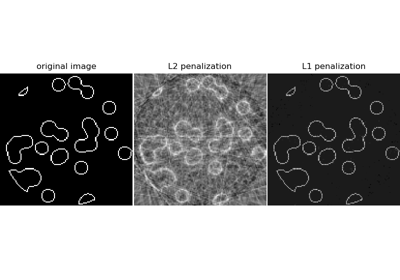Compressive sensing: tomography reconstruction with L1 prior (Lasso)

Compressive sensing: tomography reconstruction with L1 prior (Lasso)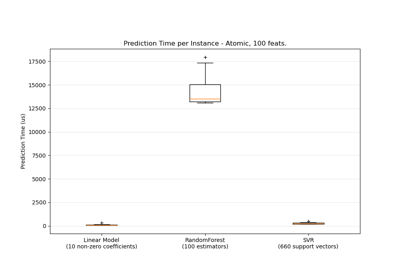Prediction Latency

Prediction Latency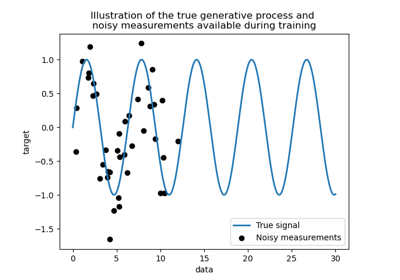Comparison of kernel ridge and Gaussian process regression

Comparison of kernel ridge and Gaussian process regressionHuberRegressor vs Ridge on dataset with strong outliers

HuberRegressor vs Ridge on dataset with strong outliersOrdinary Least Squares and Ridge Regression Variance

Ordinary Least Squares and Ridge Regression Variance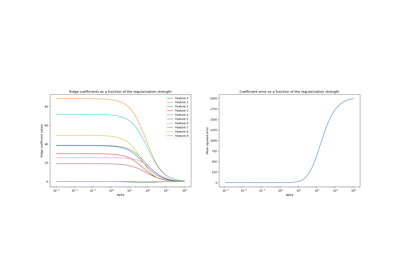Plot Ridge coefficients as a function of the L2 regularization

Plot Ridge coefficients as a function of the L2 regularization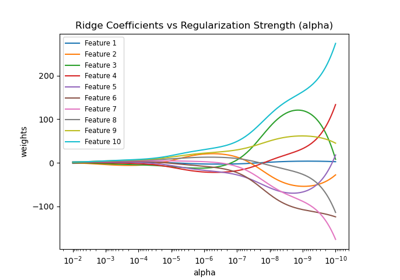Plot Ridge coefficients as a function of the regularization

Plot Ridge coefficients as a function of the regularization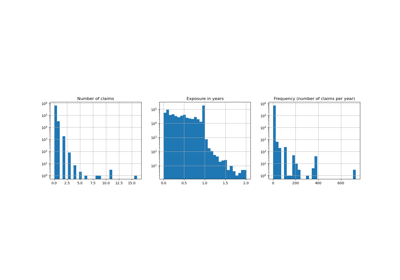Poisson regression and non-normal loss

Poisson regression and non-normal loss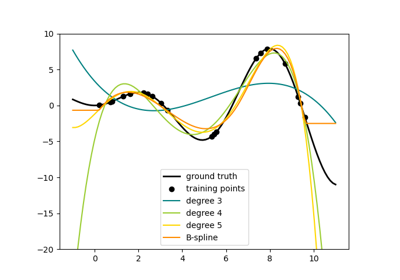Polynomial and Spline interpolation

Polynomial and Spline interpolation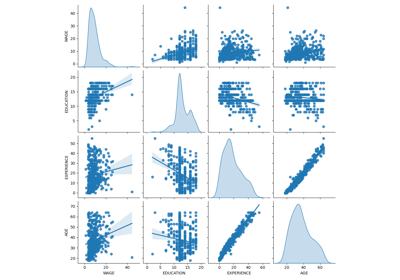Common pitfalls in the interpretation of coefficients of linear models

Common pitfalls in the interpretation of coefficients of linear models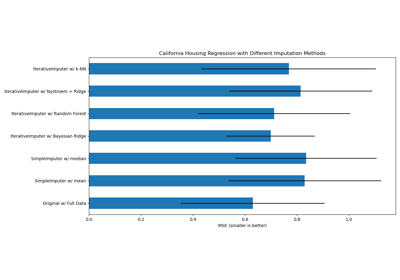Imputing missing values with variants of IterativeImputer

Imputing missing values with variants of IterativeImputer